Checkout JEE MAINS 2022 Question Paper Analysis : Checkout JEE MAINS 2022 Question Paper Analysis :

# Magnetization and Magnetic Intensity

Magnetization, also termed magnetic polarization, is a vector quantity that measures the density of permanent or induced dipole moment in a given magnetic material. As we know, magnetization results from the magnetic moment, which results from the motion of electrons in the atoms or the spin of electrons or the nuclei. The net magnetization results from the response of a material to the external magnetic field, together with any unbalanced magnetic dipole moment that is inherent in the material due to the motion in its electrons, as mentioned earlier. The concept of magnetization helps us in classifying the materials based on their magnetic property. In this section, we will learn more about magnetization and the concept of magnetic intensity.

## What is Magnetization?

The magnetization of a given sample material M can be defined as the net magnetic moment for that material per unit volume.
Mathematically,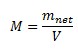Let us now consider the case of a solenoid. Let us take a solenoid with n turns per unit length and the current passing through it be given by I, then the magnetic field in the interior of the solenoid can be given as,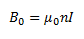Now, if we fill the interior with the solenoid with a material of non-zero magnetization, the field inside the solenoid must be greater than before. The net magnetic field B inside the solenoid can be given as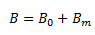Where Bm gives the field contributed by the core material. Here, Bm is proportional to the magnetization of the material, M. Mathematically,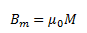Here, µ0 is the constant of permeability of a vacuum.
Let us now discuss another concept here: a material’s magnetic intensity. The magnetic intensity of a material can be given as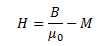From this equation, we see that the total magnetic field can also be defined as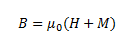Here, the magnetic field due to the external factors such as the current in the solenoid is given as H and that due to the nature of the core is given by M. The latter quantity, that is, M, is dependent on external influences and is given by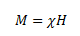Where χ is the magnetic susceptibility of the material. It measures the response of a material to an external field. The magnetic susceptibility of a material is small and positive for paramagnetic materials and is small and negative for diamagnetic materials.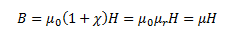Here, the term µr is termed as the relative magnetic permeability of a material, which is analogous to the dielectric constants in the case of electrostatics. We define the magnetic permeability as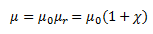## Do you know what a compass is and why do the needles in a compass move the way they move? Watch the video to find answers to these questions!## Frequently Asked Questions – FAQs

### Is magnetization a scalar or vector quantity?

Magnetization is a vector quantity.

### Does magnetization helps in classifying the materials on the basis of their magnetic property?

Yes, magnetization helps in classifying materials based on magnetic properties.

### What is the magnetic susceptibility of paramagnetic material?

Paramagnetic materials have positive magnetic susceptibility.

### Define magnetization.

Magnetization is the parameter that defines the density of induced or permanent magnetic dipole moments in a magnetic material.

True.